# Chapter 10  Objects

As we learned in the previous chapter, an object is a collection of data that provides a set of methods. For example, a String is a collection of characters that provides methods like charAt and substring.

Java is an “object-oriented” language, which means that it uses objects to represent data and provide methods related to them. This way of organizing programs is a powerful design concept, and we will introduce it a little at a time throughout the remainder of the book.

In this chapter, we introduce two new types of objects: Point and Rectangle. We show how to write methods that take objects as parameters and produce objects as return values. We also take a look at the source code for the Java library.

## 10.1  Point objects

The java.awt package provides a class named Point intended to represent the coordinates of a location in a Cartesian plane. In mathematical notation, points are often written in parentheses with a comma separating the coordinates. For example, (0,0) indicates the origin, and (x,y) indicates the point x units to the right and y units up from the origin.

In order to use the Point class, you have to import it:

 import java.awt.Point;

Then, to create a new point, you have to use the new operator:

 Point blank; blank = new Point(3, 4);

The first line declares that blank has type Point. The second line creates the new Point with the given arguments as coordinates.

The result of the new operator is a reference to the new object. So blank contains a reference to the new Point object. Figure 10.1 shows the result.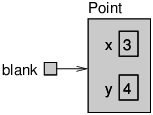Figure 10.1: State diagram showing a variable that refers to a Point object.

As usual, the name of the variable blank appears outside the box, and its value appears inside the box. In this case, the value is a reference, which is represented with an arrow. The arrow points to the new object, which contains two variables, x and y.

## 10.2  Attributes

Variables that belong to an object are usually called attributes, but you might also see them called “fields”. To access an attribute of an object, Java uses dot notation. For example:

 int x = blank.x;

The expression blank.x means “go to the object blank refers to, and get the value of the attribute x.” In this case, we assign that value to a local variable named x. There is no conflict between the local variable named x and the attribute named x. The purpose of dot notation is to identify which variable you are referring to unambiguously.

You can use dot notation as part of an expression. For example:

 System.out.println(blank.x + ", " + blank.y); int sum = blank.x * blank.x + blank.y * blank.y;

The first line displays 3, 4; the second line calculates the value 25.

## 10.3  Objects as parameters

You can pass objects as parameters in the usual way. For example:

 public static void printPoint(Point p) { System.out.println("(" + p.x + ", " + p.y + ")"); }

This method takes a point as an argument and displays its attributes in parentheses. If you invoke printPoint(blank), it displays (3, 4).

But we don’t really need a method like printPoint, because if you invoke System.out.println(blank) you get:

 java.awt.Point[x=3,y=4]

Point objects provide a method called toString that returns a string representation of a point. When you call println with objects, it automatically calls toString and displays the result. In this case, it shows the name of the type (java.awt.Point) and the names and values of the attributes.

As another example, we can rewrite the distance method from Section 6.2 so that it takes two Points as parameters instead of four doubles.

 public static double distance(Point p1, Point p2) { int dx = p2.x - p1.x; int dy = p2.y - p1.y; return Math.sqrt(dx * dx + dy * dy); }

Passing objects as parameters makes the source code more readable and less error-prone, because related values are bundled together.

## 10.4  Objects as return types

The java.awt package also provides a class called Rectangle. To use it, you have to import it:

 import java.awt.Rectangle;

Rectangle objects are similar to points, but they have four attributes: x, y, width, and height. The following example creates a Rectangle object and makes the variable box refer to it:

 Rectangle box = new Rectangle(0, 0, 100, 200);

Figure 10.2 shows the effect of this assignment.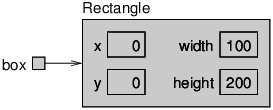Figure 10.2: State diagram showing a Rectangle object.

If you run System.out.println(box), you get:

 java.awt.Rectangle[x=0,y=0,width=100,height=200]

Again, println uses the toString method provided by Rectangle, which knows how to display Rectangle objects.

You can write methods that return objects. For example, findCenter takes a Rectangle as an argument and returns a Point with the coordinates of the center of the rectangle:

 public static Point findCenter(Rectangle box) { int x = box.x + box.width / 2; int y = box.y + box.height / 2; return new Point(x, y); }

The return type of this method is Point. The last line creates a new Point object and returns a reference to it.

## 10.5  Mutable objects

You can change the contents of an object by making an assignment to one of its attributes. For example, to “move” a rectangle without changing its size, you can modify the x and y values:

 Rectangle box = new Rectangle(0, 0, 100, 200); box.x = box.x + 50; box.y = box.y + 100;

The result is shown in Figure 10.3.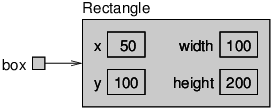Figure 10.3: State diagram showing updated attributes.

We can encapsulate this code in a method and generalize it to move the rectangle by any amount:

 public static void moveRect(Rectangle box, int dx, int dy) { box.x = box.x + dx; box.y = box.y + dy; }

The variables dx and dy indicate how far to move the rectangle in each direction. Invoking this method has the effect of modifying the Rectangle that is passed as an argument.

 Rectangle box = new Rectangle(0, 0, 100, 200); moveRect(box, 50, 100); System.out.println(box);

Modifying objects by passing them as arguments to methods can be useful. But it can also make debugging more difficult, because it is not always clear which method invocations modify their arguments.

Java provides a number of methods that operate on Points and Rectangles. For example, translate has the same effect as moveRect, but instead of passing the rectangle as an argument, you use dot notation:

 box.translate(50, 100);

This line invokes the translate method for the object that box refers to. As a result, the box object is updated directly.

This example is a good illustration of object-oriented programming. Rather than write methods like moveRect that modify one or more parameters, we apply methods to objects themselves using dot notation.

## 10.6  Aliasing

Remember that when you assign an object to a variable, you are assigning a reference to an object. It is possible to have multiple variables that refer to the same object. The state diagram in Figure 10.4 shows the result.

 Rectangle box1 = new Rectangle(0, 0, 100, 200); Rectangle box2 = box1;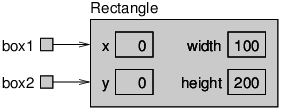Figure 10.4: State diagram showing two variables that refer to the same object.

Notice how box1 and box2 are aliases for the same object, so any changes that affect one variable also affect the other. This example adds 50 to all four sides of the rectangle, so it moves the corner up and to the left by 50, and it increases the height and width by 100:

 System.out.println(box2.width); box1.grow(50, 50); System.out.println(box2.width);

The first line displays 100, which is the width of the Rectangle referred to by box2. The second line invokes the grow method on box1, which stretches the Rectangle horizontally and vertically. The effect is shown in Figure 10.5.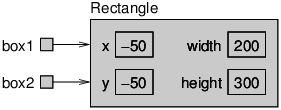Figure 10.5: State diagram showing the effect of invoking grow.

When we make a change using box1, we see the change using box2. Thus, the value displayed by the third line is 200, the width of the expanded rectangle.

## 10.7  The null keyword

When you create an object variable, remember that you are storing a reference to an object. In Java, the keyword null is a special value that means “no object”. You can declare and initialize object variables this way:

 Point blank = null;

The value null is represented in state diagrams by a small box with no arrow, as in Figure 10.6.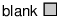Figure 10.6: State diagram showing a variable that contains a null reference.

If you try to use a null value, either by accessing an attribute or invoking a method, Java throws a NullPointerException.

 Point blank = null; int x = blank.x; // NullPointerException blank.translate(50, 50); // NullPointerException

On the other hand, it is legal to pass a null reference as an argument or receive one as a return value. For example, null is often used to represent a special condition or indicate an error.

## 10.8  Garbage collection

In Section 10.6, we saw what happens when more than one variable refers to the same object. What happens when no variables refer to an object?

 Point blank = new Point(3, 4); blank = null;

The first line creates a new Point object and makes blank refer to it. The second line changes blank so that instead of referring to the object, it refers to nothing. In the state diagram, we remove the arrow between them, as in Figure 10.7.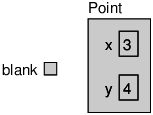Figure 10.7: State diagram showing the effect of setting a variable to null.

If there are no references to an object, there is no way to access its attributes or invoke a method on it. From the programmer’s view, it ceases to exist. However it’s still present in the computer’s memory, taking up space.

As your program runs, the system automatically looks for stranded objects and reclaims them; then the space can be reused for new objects. This process is called garbage collection.

You don’t have to do anything to make garbage collection happen, and in general don’t have to be aware of it. But in high-performance applications, you may notice a slight delay every now and then when Java reclaims space from discarded objects.

## 10.9  Class diagrams

To summarize what we’ve learned so far, Point and Rectangle objects each have their own attributes and methods. Attributes are an object’s data, and methods are an object’s code. An object’s class defines which attributes and methods it will have.

In practice, it’s more convenient to look at high-level pictures than to examine the source code. Unified Modeling Language (UML) defines a standard way to summarize the design of a class.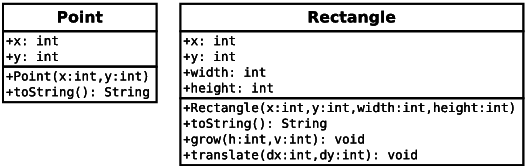Figure 10.8: UML class diagrams for Point and Rectangle.

As shown in Figure 10.8, a class diagram is divided into two sections. The top half lists the attributes, and the bottom half lists the methods. UML uses a language-independent format, so rather than showing int x, the diagram uses x: int.

In contrast to state diagrams, which visualize objects (and variables) at run-time, a class diagram visualizes the source code at compile-time.

Both Point and Rectangle have additional methods; we are only showing the ones introduced in this chapter. See the documentation for these classes to learn more about what they can do.

## 10.10  Java library source

Throughout the book, you have used classes from the Java library including System, String, Scanner, Math, Random, and others. You may not have realized that these classes are written in Java. In fact, you can take a look at the source code to see how they work.

The Java library contains thousands of files, many of which are thousands of lines of code. That’s more than one person could read and understand fully, so please don’t be intimidated!

Because it’s so large, the library source code is stored in a file named src.zip. Take a few minutes to locate this file on your machine:

• On Linux, it’s likely under: /usr/lib/jvm/openjdk-8/
(You might need to install the openjdk-8-source package.)
• On OS X, it’s likely under:
/Library/Java/JavaVirtualMachines/jdk.../Contents/Home/
• On Windows, it’s likely under: C:\Program Files\Java\jdk...\

When you open (or unzip) the file, you will see folders that correspond to Java packages. For example, open the java folder and then open the awt folder. You should now see Point.java and Rectangle.java, along with the other classes in the java.awt package.

Open Point.java in your editor and skim through the file. It uses language features we haven’t yet discussed, so you probably won’t understand everything. But you can get a sense of what professional Java software looks like by browsing through the library.

Notice how much of Point.java is documentation. Each method is thoroughly commented, including @param, @return, and other Javadoc tags. Javadoc reads these comments and generates documentation in HTML. You can see the results by reading the documentation for the Point class, which you can find by doing a web search for “Java Point”.

Now take a look at Rectangle’s grow and translate methods. There is more to them than you may have realized, but that doesn’t limit your ability to use these methods in a program.

To summarize the whole chapter, objects encapsulate data and provide methods to access and modify the data directly. Object-oriented programming makes it possible to hide messy details so that you can more easily use and understand code that other people wrote.

## 10.11  Vocabulary

attribute:
One of the named data items that make up an object.
dot notation:
Use of the dot operator (.) to access an object’s attributes or methods.
object-oriented:
A way of organizing code and data into objects, rather than independent methods.
garbage collection:
The process of finding objects that have no references and reclaiming their storage space.
UML:
Unified Modeling Language, a standard way to draw diagrams for software engineering.
class diagram:
An illustration of the attributes and methods for a class.

## 10.12  Exercises

The code for this chapter is in the ch10 directory of ThinkJavaCode. See page ?? for instructions on how to download the repository. Before you start the exercises, we recommend that you compile and run the examples.

Exercise 1   The point of this exercise is to make sure you understand the mechanism for passing objects as parameters.
1. For the following program, draw a stack diagram showing the local variables and parameters of main and riddle just before riddle returns. Use arrows to show which objects each variable references.
2. What is the output of the program?
3. Is the blank object mutable or immutable? How can you tell?
 public static int riddle(int x, Point p) { x = x + 7; return x + p.x + p.y; }
 public static void main(String[] args) { int x = 5; Point blank = new Point(1, 2); System.out.println(riddle(x, blank)); System.out.println(x); System.out.println(blank.x); System.out.println(blank.y); }
Exercise 2   The point of this exercise is to make sure you understand the mechanism for returning new objects from methods.
1. Draw a stack diagram showing the state of the program just before distance returns. Include all variables and parameters, and show the objects those variables refer to.
2. What is the output of this program? (Can you tell without running it?)
 public static double distance(Point p1, Point p2) { int dx = p2.x - p1.x; int dy = p2.y - p1.y; return Math.sqrt(dx * dx + dy * dy); } public static Point findCenter(Rectangle box) { int x = box.x + box.width / 2; int y = box.y + box.height / 2; return new Point(x, y); }
 public static void main(String[] args) { Point blank = new Point(5, 8); Rectangle rect = new Rectangle(0, 2, 4, 4); Point center = findCenter(rect); double dist = distance(center, blank); System.out.println(dist); }
Exercise 3   This exercise is about aliasing. Recall that aliases are two variables that refer to the same object.
1. Draw a diagram that shows the state of the program just before the end of main. Include all local variables and the objects they refer to.
2. What is the output of the program?
3. At the end of main, are p1 and p2 aliased? Why or why not?
 public static void printPoint(Point p) { System.out.println("(" + p.x + ", " + p.y + ")"); } public static Point findCenter(Rectangle box) { int x = box.x + box.width / 2; int y = box.y + box.height / 2; return new Point(x, y); }
 public static void main(String[] args) { Rectangle box1 = new Rectangle(2, 4, 7, 9); Point p1 = findCenter(box1); printPoint(p1); box1.grow(1, 1); Point p2 = findCenter(box1); printPoint(p2); }
Exercise 4

You might be sick of the factorial method by now, but we’re going to do one more version.

1. Create a new program called Big.java and write (or reuse) an iterative version of factorial.
2. Display a table of the integers from 0 to 30 along with their factorials. At some point around 15, you will probably see that the answers are not right anymore. Why not?

3. BigInteger is a Java class that can represent arbitrarily big integers. There is no upper bound except the limitations of memory size and processing speed. Take a minute to read the documentation, which you can find by doing a web search for “Java BigInteger”.
4. To use BigIntegers, you have to import java.math.BigInteger at the beginning of your program.
5. There are several ways to create a BigInteger, but the simplest uses valueOf. The following code converts an integer to a BigInteger:
 int x = 17; BigInteger big = BigInteger.valueOf(x);
6. Since BigIntegers are not primitive types, the usual math operators don’t work. Instead, we have to use methods like add. To add two BigIntegers, invoke add on one and pass the other as an argument.
 BigInteger small = BigInteger.valueOf(17); BigInteger big = BigInteger.valueOf(1700000000); BigInteger total = small.add(big);

Try out some of the other methods, like multiply and pow.

7. Convert factorial so that it performs its calculation using BigIntegers and returns a BigInteger as a result. You can leave the parameter alone; it will still be an integer.
8. Try displaying the table again with your modified factorial method. Is it correct up to 30? How high can you make it go?
9. Are BigInteger objects mutable or immutable? How can you tell?
Exercise 5   Many encryption algorithms depend on the ability to raise large integers to a power. Here is a method that implements an efficient algorithm for integer exponentiation:
 public static int pow(int x, int n) { if (n == 0) return 1; // find x to the n/2 recursively int t = pow(x, n / 2); // if n is even, the result is t squared // if n is odd, the result is t squared times x if (n % 2 == 0) { return t * t; } else { return t * t * x; } }

The problem with this method is that it only works if the result is small enough to be represented by an int. Rewrite it so that the result is a BigInteger. The parameters should still be integers, though.

You should use the BigInteger methods add and multiply. But don’t use BigInteger.pow; that would spoil the fun.

Text © Allen Downey and Chris Mayfield. Interactive HTML © Trinket. Both provided under a CC-NC-BY license. Think Java 1st Edition, Version 6.1.3. 2nd Edition available here.